# Relaxation Oscillator: What is it? (And How Does it Work)

Contents

## What is a Relaxation Oscillator?

A relaxation oscillator is defined as a non-linear electronic oscillator circuit that can generate a non-sinusoidal repetitive output signal. A relaxation oscillator was invented by Henri Abraham and Eugene Bloch using a vacuum tube during World War 1.

Oscillators are classified into two different categories; linear oscillators (for sinusoidal waveforms) and relaxation oscillators (for non-sinusoidal waveforms).

It must provide a repetitive and periodic signal for non-sinusoidal waveforms like triangular, square, and rectangular waves at its output.

The design of the relaxation oscillator must include non-linear elements like the transistor, Op-Amp, or MOSFET and energy storing devices like capacitor and inductor.

To produce a cycle, the capacitor and inductor charge and discharge continuously. And the frequency of the cycle or period of oscillation depends on the time constant.

## How Does a Relaxation Oscillator Work?

The relaxation oscillator contains energy storing devices like capacitor and inductor. These devices are charged by a source and discharge through a load.

The shape of the output waveform of the relaxation oscillator depends on the time constant of the circuit.

Let’s understand the working of the relaxation oscillators with an example.

Here, a capacitor is connected between a bulb and a battery. This circuit is also known as the flasher circuit or RC relaxation oscillator.

A battery charges the capacitor through the resistor. During charging of the capacitor, the bulb remains in OFF condition.

When the capacitor reaches its threshold value, it discharges through the bulb. Thus, during discharging of the capacitor, the bulb is glowing.

When the capacitor is discharged, it starts charging by the source again. And the bulb remains OFF.

So, the process of charging and discharging the capacitor is continuous and periodic.

The charging time of the capacitor determines by the time constant. And the time constant depends on the value of the resistor and capacitor for the RC circuit.

Therefore, the flashing rate of the bulb decides by the value of the resistor and capacitor.

The waveforms across the bulb are as shown in the figure below.

To control the output waveform, non-linear elements are used in the circuit.

## Relaxation Oscillator Circuit Diagram

The relaxation oscillator circuit diagram contains a non-linear device to generate different types of the output waveform. According to the use of non-linear devices, the relaxation oscillator classifies three types of circuit diagrams.

### Op-Amp Relaxation Oscillator

An op-Amp relaxation oscillator is also known as an astable multivibrator. It is used to generate square waves. The circuit diagram of the Op-Amp relaxation oscillator is shown in the figure below.

This circuit contains a capacitor, resistors, and an Op-Amp.

The non-inverting terminal of Op-Amp is connected with an RC circuit. So, the capacitor voltage VC is the same as the voltage at non-inverting terminal V- of Op-Amp. And inverting terminal is connected with the resistors.

When the Op-Amp is used with positive feedback, as shown in the circuit diagram, the circuit is known as the Schmitt trigger.

When V+ is greater than V-, the output voltage is +12V. And when V- is greater than V+, the output voltage is -12V.

For the initial condition, at time t=0, assume that the capacitor is fully discharged. Hence the voltage at the non-inverting terminal is V-=0. And the voltage at the inverting terminals V+ is equal to βVout.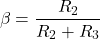To make calculation easy, we consider that R2 and R3 are the same. So, β=2 and βVout=6V. So, the capacitor will charge and discharge up to 6V.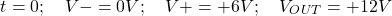In this condition, V+ is greater than V-. So, output voltage Vout=+12V. And the capacitor starts charging.

When the capacitor voltage is greater than 6V, V- is greater than V+. Hence, the output voltage changes to -12V.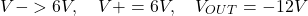During this condition, the inverting terminal voltage changes its polarity. So, V+=-6V.

Now, the capacitor discharges up to -6V. When the capacitor voltage is less than -6V, again V+ is greater than V-.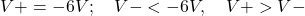Therefore, again the output voltage changes -12V to +12V. And again, the capacitor starts charging.

So, the charging and discharging cycle of the capacitor generates a periodic and repetitive square wave at the output terminal, as shown in the figure below.

The frequency of the output waveform depends on the charging and discharging time of the capacitor. And the charging-discharging time of the capacitor depends on the time constant of the RC circuit.

### UJT Relaxation Oscillator

UJT (unijunction transistor) is used as a switching device in the relaxation oscillator. The circuit diagram of the UJT relaxation oscillator is shown in the figure below.

The emitter terminal of UJT is connected with a resistor and a capacitor.

We assume that initially the capacitor is discharged. So, the capacitor voltage is zero.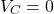In this condition, UJT remains OFF. And the capacitor starts charging through the resistor R by the equation below.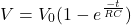The capacitor continues to charge until it reaches the maximum supplied voltage VBB.

When the voltage across the capacitor is greater than the supplied voltage, it enables the UJT turned ON. Then the capacitor stops charging and starts discharging through the resistor R1.

The capacitor continues to discharge until the capacitor voltage reaches the valley voltage (VV) of UJT. After that, the UJT turned OFF and started the capacitor charging.

Hence, the process of charging and discharging the capacitor generates a saw-tooth waveform across the capacitor. And the voltage appears across the resistor R2 during discharging of the capacitor and remains zero during charging of the capacitor.

The voltage waveform across the capacitor and resistor R2 is shown in the figure below.

## Relaxation Oscillator Frequency

The frequency of the Relaxation Oscillator depends on the charging and discharging time of the capacitor. In the RC circuit, the charging and discharging time decides by the time constant.

### Frequency of Op-Amp Relaxation Oscillator

In the Op-Amp relaxation oscillator, the R1 and C1 contribute to the frequency of oscillation. Therefore, for lower frequency oscillation, we need a longer time for charging and discharging the capacitor. And for a long time of charging and discharging, we need to set a more significant R1 and C1.

Similarly, a smaller value of R1 and C1 causes higher frequency oscillation.

But, in the calculation of frequency, resistor R2 and R3 also play a vital role. Because these resistors will decide the threshold voltage of the capacitor, and the capacitor will charge up to this voltage level.

Suppose the threshold voltage is lower, the charging time is faster. Similarly, the threshold voltage is higher; the charging time is slower.

Therefore, the oscillation frequency depends on the value of R1, R2, R3, and C1. And Op-Amp relaxation oscillator frequency formula is;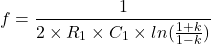Where,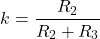In most of the conditions, R2 and R3 are the same to make easy design and calculation.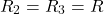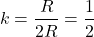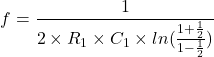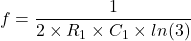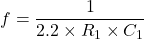By putting the values of R1 and C1, we can find the oscillation frequency of the Op-Amp relaxation oscillator.

### Frequency of UJT Relaxation Oscillator

In the UJT relaxation oscillator, also the frequency depends on the RC circuit. As shown in the circuit diagram of the UJT relaxation oscillator, resistors R1 and R2 are current limiting resistors. And the frequency of oscillation depends on the resistor R and capacitor C.

The formula of frequnecy for UJT relaxation oscillator is;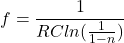Where;

n = Intrinsic stand-off ratio. And the value of n lies between 0.51 to 0.82.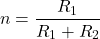To turn ON the UJT, minimum voltage required is;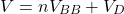Where,

VBB = supply voltage

VD = internal diode drop between emitter and base-2 terminal

The value of resistor R limits between following range.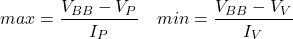Where,

VP, IP = peak voltage and current

VV, IV = valley voltage and current

## Relaxation Oscillator Differential Equation

In the circuit diagram of the relaxation oscillator, resistors R2 and R3 have equal values. So, according to the voltage divider rule;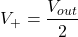V is obtained by ohm’s law and capacitor differential equation;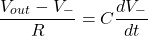There are two solutions to this differential equation; particular solution and homogenous solution.

For a particular solution, V- is a constant. Assume V = A. Therefore, the differentiation of constant is zero,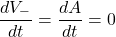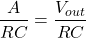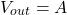For homogenous solution, use the Laplace transform of below equation;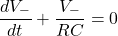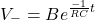V is the total of particular and homogenous solutions.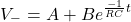To find the value of B, we required to evaluate the initial condition.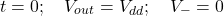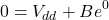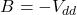So, final solution of V- is;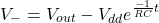## Comparator vs Op-Amps

A comparator is also used instead of Op-Amp. Similar to Op-Amp, the compensators are designed to be driven rail-to-rail.

The comparator has a faster rise time and fall time compared to Op-Amp. Therefore, the comparator is more suitable than the Op-Amp for the oscillator circuit.

In the case of the Op-Amp, it has push-pull outputs. So, if you are using Op-Amp, it is not necessary to use a pull-up resistor. But if you are using a comparator, it must use a pull-up resistor.

## Applications of Relaxation Oscillators

The relaxation oscillators are used to generate an internal clock signal for any digital circuit. It is also used in the applications listed below.

• Voltage control oscillator
• Memory circuits
• Signal generator (to generate clock signals)
• Stroboscopes
• Firing thyristor-based circuit
• Multi-vibrators